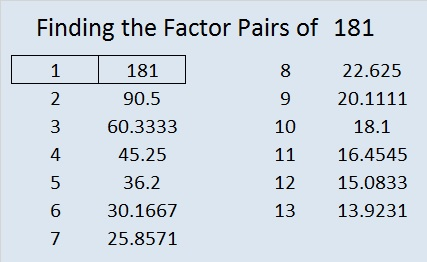# 181 and Level 2

• 181 is a prime number.
• Prime factorization: 181 is prime.
• The exponent of prime number 181 is 1. Adding 1 to that exponent we get (1 + 1) = 2. Therefore 181 has exactly 2 factors.
• Factors of 181: 1, 181
• Factor pairs: 181 = 1 x 181
• 181 has no square factors that allow its square root to be simplified. √181 ≈ 13.45362How do we know that 181 is a prime number? If 181 were not a prime number, then it would be divisible by at least one prime number less than or equal to √181 ≈ 13.5. Since 181 cannot be divided evenly by 2, 3, 5, 7, 11, or 13, we know that 181 is a prime number.Excel file of puzzles and previous week’s factor solutions: 10 Factors 2014-07-21This site uses Akismet to reduce spam. Learn how your comment data is processed.